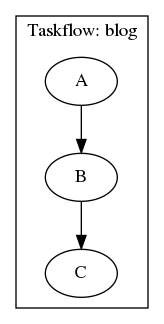### Manoj Rao

In earlier posts I wrote about experimenting with C++ Taskflow. While it is an excellent library for incorporating task based programming into your project it lacks an important feature of enabling directed data flow much like its control flow.

Consider the fofollowing example:

``````tf::Taskflow taskflow;

auto input_a = 0, input_b = 1, input_c = 2;
int result_a = 0, result_b = 0, result_c;
auto [A, B, C] = taskflow.emplace(
[&input_a](){ std::cout << "Task A\n"; result_a = produce_result(input_a); },
[&input_b](){ std::cout << "Task B\n"; result_b = produce_result(input_b); },
[&input_c](){ std::cout << "Task C\n"; result_c = produce_result(input_c); }
);
``````

Basically, Taskflow does not allow for `result_a` to be fed into Task B in place of `input_b`. Similarly, does not allow for `result_b` to be fed into Task C in place of `input_c`.

``````auto [A, B, C] = taskflow.emplace(
[&input_a](){ std::cout << "Task A\n"; result_a = produce_result(input_a); },
[&input_b](){ std::cout << "Task B\n"; result_b = produce_result(result_a); },
[&input_c](){ std::cout << "Task C\n"; result_c = produce_result(result_b); }
);
``````

On browsing through the issues on Github I found many others have already requested for this ability. But I was hoping for a better response. In any case, the author makes sense that there are going to be many ways in which we can accomplish this.

In any case, achieving control flow is the harder part. If, I understand the response above I think what they are implying is the following:

``````auto [A, B, C] = taskflow.emplace(
[&input_a](){ std::cout << "Task A\n"; result_a = produce_result(input_a); },
[&result_a](){ std::cout << "Task B\n"; result_b = produce_result(result_a); },
[&result_b](){ std::cout << "Task C\n"; result_c = produce_result(result_b); }
);
``````

This will work as long as the task graph is structured in a way that `A -> B -> C`. To do this, we can use the following:

``````A.precede(B);
B.precede(C);
``````

Therefore, the final version of this code will look like:

``````

auto input_a = 0, input_b = 1, input_c = 2;
int result_a = 0, result_b = 0, result_c;
auto [A, B, C] = taskflow.emplace(
[&input_a](){ std::cout << "Task A\n"; result_a = produce_result(input_a); },
[&result_a](){ std::cout << "Task B\n"; result_b = produce_result(result_a); },
[&result_b](){ std::cout << "Task C\n"; result_c = produce_result(result_b); }
);

A.precede(B);
B.precede(C);

``````

This results in the following output:#### My Podcast!

If you like topics such as this then please consider subscribing to my podcast. I talk to some of the stalwarts in tech and ask them what their favorite productivity hacks are:

##### Available on iTunes Podcast